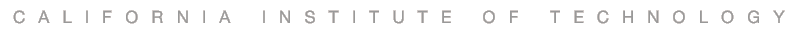# THE LECTURES

• Taught by Feynman Prize winner Professor Yaser Abu-Mostafa.
• The fundamental concepts and techniques are explained in detail. The focus of the lectures is real understanding, not just "knowing."
• Lectures use incremental viewgraphs (2853 in total) to simulate the pace of blackboard teaching.
• The 18 lectures (below) are available on different platforms:

Here is the playlist on YouTube

Lectures are available on iTunes U course app

Place the mouse on a lecture title for a short description

• Lecture 1 (The Learning Problem)
Lecture (some audio drops, sorry!) - Q&A - Slides
• The Learning Problem - Introduction; supervised, unsupervised, and reinforcement learning. Components of the learning problem.

• Lecture 2 (Is Learning Feasible?)
Review - Lecture - Q&A - Slides
• Is Learning Feasible? - Can we generalize from a limited sample to the entire space? Relationship between in-sample and out-of-sample.

• Lecture 3 (The Linear Model I)
Review - Lecture - Q&A - Slides
• The Linear Model I - Linear classification and linear regression. Extending linear models through nonlinear transforms.

• Lecture 4 (Error and Noise)
Review - Lecture - Q&A - Slides
• Error and Noise - The principled choice of error measures. What happens when the target we want to learn is noisy.

• Lecture 5 (Training versus Testing)
Review - Lecture - Q&A - Slides
• Training versus Testing - The difference between training and testing in mathematical terms. What makes a learning model able to generalize?

• Lecture 6 (Theory of Generalization)
Review - Lecture - Q&A - Slides
• Theory of Generalization - How an infinite model can learn from a finite sample. The most important theoretical result in machine learning.

• Lecture 7 (The VC Dimension)
Review - Lecture - Q&A - Slides
• The VC Dimension - A measure of what it takes a model to learn. Relationship to the number of parameters and degrees of freedom.

Review - Lecture - Q&A - Slides
• Bias-Variance Tradeoff - Breaking down the learning performance into competing quantities. The learning curves.

• Lecture 9 (The Linear Model II)
Review - Lecture - Q&A - Slides
• The Linear Model II - More about linear models. Logistic regression, maximum likelihood, and gradient descent.

• Lecture 10 (Neural Networks)
Review - Lecture - Q&A - Slides
• Neural Networks - A biologically inspired model. The efficient backpropagation learning algorithm. Hidden layers.

• Lecture 11 (Overfitting)
Review - Lecture - Q&A - Slides
• Overfitting - Fitting the data too well; fitting the noise. Deterministic noise versus stochastic noise.

• Lecture 12 (Regularization)
Review - Lecture - Q&A - Slides
• Regularization - Putting the brakes on fitting the noise. Hard and soft constraints. Augmented error and weight decay.

• Lecture 13 (Validation)
Review - Lecture - Q&A - Slides
• Validation - Taking a peek out of sample. Model selection and data contamination. Cross validation.

• Lecture 14 (Support Vector Machines)
Review - Lecture - Q&A - Slides
• Support Vector Machines - One of the most successful learning algorithms; getting a complex model at the price of a simple one.

• Lecture 15 (Kernel Methods)
Review - Lecture - Q&A - Slides
• Kernel Methods - Extending SVM to infinite-dimensional spaces using the kernel trick, and to non-separable data using soft margins.

• Lecture 16 (Radial Basis Functions)
Review - Lecture - Q&A - Slides
• Radial Basis Functions - An important learning model that connects several machine learning models and techniques.

• Lecture 17 (Three Learning Principles)
Review - Lecture - Q&A - Slides
• Three Learning Principles - Major pitfalls for machine learning practitioners; Occam's razor, sampling bias, and data snooping.

• Lecture 18 (Epilogue)
Review - Lecture - Acknowledgment - Slides
• Epilogue - The map of machine learning. Brief views of Bayesian learning and aggregation methods.